# Thin Lenses

### Guest Account

Attempt Form Four Physics Questions
Guest Account

# Thin Lenses

A lens is conventionally defined as a piece of glass which is used to focus or change the direction of a beam of light passing through it.
They are mainly made of glass or plastic. Lens are used in making spectacles, cameras, cinema projectors, microscopes and telescopes.

## Types of Thin Lenses

A lens which is thicker at its centre than at its edges converges light and is called convex or converging lens. A lens which is thicker at its edges than at its centre diverges light and is known as concave or diverging lens.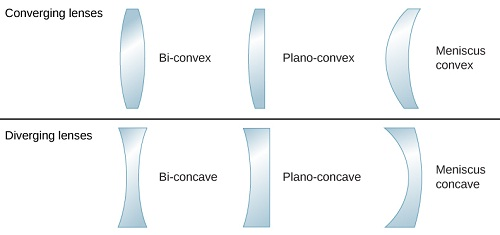### Properties of lenses.

1. Optical centre This is the geometric centre of a lens which is usually shown using a black dot in ray diagrams. A ray travelling through the optical centre passes through in a straight line.
2. Centre of curvature – this is the geometric centre of the circle of which the lens surface is part of. Since lenses have two surfaces there are two centres of curvature. C is used to denote one centre while the other is denoted by C1
3. Principal axis this is an imaginary line which passes through the optical centre at right angle to the lens.
4. Principal focus this is a point through which all rays travelling parallel to the principal axis pass after refraction through the lens. A lens has a principal focus on both its sides. F is used to denote the principal focus
5. Focal length this is the distance between the optical centre and the principal focus. It is denoted by ‘f’.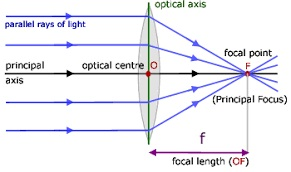The principal focus for a converging lens is real and virtual for a diverging lens.
It is important to note that the principal focus is not always halfway between the optical centre and the centre of curvature as it is in mirrors.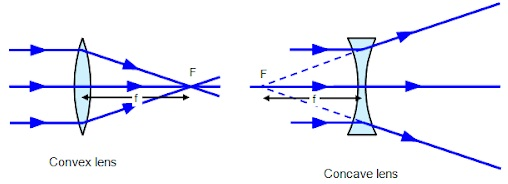### Images Formed by Thin Lenses.

The nature, size and position of the image formed by a particular lens depends on the position of the object in relation to the lens.

#### Construction of ray diagrams

Three rays are of particular importance in the construction of ray diagrams
1. A ray of light travelling parallel to the principal axis passes through the principal focus on refraction through the lens. In case of a concave lens the ray is diverged in a way that it appears to come from the principal focus.
2. A ray of light travelling through the optical centre goes un-deviated along the same path.
3. A ray of light travelling through the principal focus is refracted parallel to the principal axis on passing through the lens. The construction of the rays is illustrated below.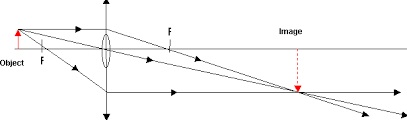### Images formed by a converging lens.

1. Object between the lens and the principal focus.
• Image formed behind the object
• Virtual
• Erect
• Magnified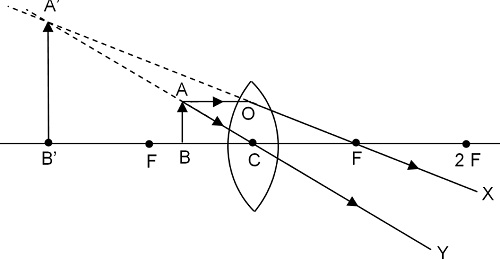2. Object at Infinity
• Image formed at the principal focus of the lens
• Real
• Inverted
• Diminished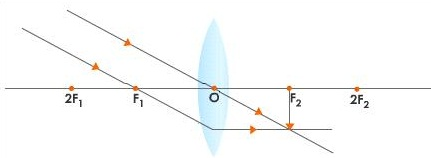3. Object at the principal focus (at F).
• Image is at infinity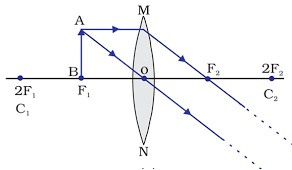4. Object between the principal focus (F) and 2 F.
• Image situated beyond 2 F
• Real
• Inverted
• Magnified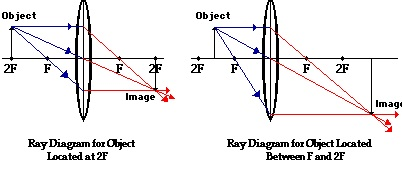5. Object at 2 F.
• Image is formed at 2 F
• Real
• Inverted
• Same size as the object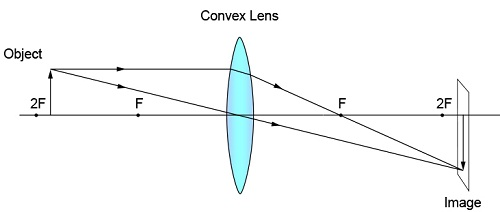6. Object beyond F.
• Image moves nearer to F as object shifts further beyond 2 F
• Real
• Inverted
• Diminished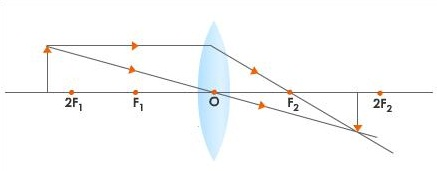### Images formed by a diverging lens

Images formed by diverging lens are always erect, virtual and diminished for all positions of the object.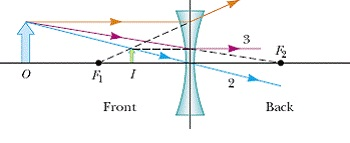#### Linear magnification.

The linear magnification produced by a lens defined as the ratio of the height of the image to
the height of the object, denoted by letter ‘m’, therefore;
m = height of the image / height of the object.
Magnification is also given by = distance of the image from the lens/ dist. of object from lens.
m = v / u
Example
An object 0.05 m high is placed 0.15 m in front of a convex lens of focal length 0.1 m. Find by construction, the position, nature and size of the image. What is the magnification?
Solution
Let 1 cm represent 5 cm. hence 0.05 m = 5 cm = 1 cm – object height
0.15 m = 15 cm = 3 cm
0.1 m = 10 cm = 2 cm – focal length.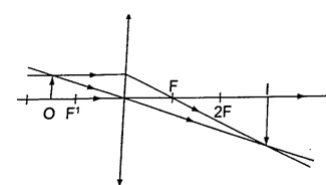• Inverted
• Real
• Magnified

### The lens formula

Let the object distance be represented by ‘u’, the image distance by ‘v’ and the focal length by ‘f’, then the general formula relating the three quantities is given by; 1 / f = 1 / u + 1 / v – this is the lens formula.

#### Examples

1. An object is placed 12 cm from a converging lens of focal length 18 cm. Find the position of the image.
Solution
Since it is a converging lens f = +18 cm (real-is-positive and virtual-is-negative rule) The object is real therefore u = +12 cm, substituting in the lens formula, then 1 / f = 1 / u + 1 / v or 1 / v = 1 / f – 1 / u = 1 / 18 – 1 / 12 = - 1 / 36 Hence v = - 36 then the image is virtual, erect and same size as the object.
2. The focal length of a converging lens is found to be 10 cm. How far should the lens be placed from an illuminated object to obtain an image which is magnified five times on the screen?
Solution
f = + 10 cm m = v / u = 5 hence v = 5 u
Using the lens formula 1 / f = 1 / u + 1 / v => 1 /10 = 1 / u + 1 / 5 u (replacing v with 5 u) 1 / 10 = 6 / 5 u, hence 5 u = 60 giving u = 12 cm (the lens should be placed 12 cm from the illuminated object)
3. The lens of a slide projector focuses on an image of height 1.5m on a screen placed 9.0 m from the projector. If the height of the picture on the slide was 6.5 cm, determine,
a) Distance from the slide (picture) to the lens
b) Focal length of the lens
Solution
Magnification = height of the image / height of the object = v / u = 150 / 6.5 = 900 / u
u = 39 cm (distance from slide to the lens). m = 23.09
1 / f = 1 / u + 1 / v = 1 /39 + 1 / 90 =
0.02564 + 0.00111
1 / f = 0.02675 (reciprocal tables) f = 37.4 cm.

### Determining focal lengths

1. Determining focal length of a converging lens
Experiment: To determine the focal length of a converging lens using the lens formula.
Procedure.
1. Set up the apparatus as shown below
2.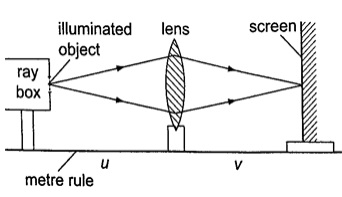3. Place the object at reasonable length from the screen until a real image is formed on the screen. Move the lens along the metre rule until a sharply focused image is obtained.
4. By changing the position of the object obtain several pairs of value of u and v and record your results as shown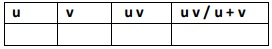#### Discussion

The value u v / u + v is the focal length of the lens and the different sets of values give the average value of ‘f’. Alternatively the value ‘f’ may be obtained by plotting a graph of 1 / v against 1 / u. When plotted the following graph is obtained.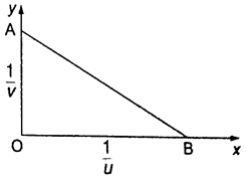Since 1 / f = 1 / u + 1 / v, at the y-intercept 1 / u = 0, so that 1/ f = 1 / v or f = v.
The focal length may therefore be obtained by reading off the y-intercept and finding the reciprocal. Similarly at the x-intercept, 1 / v = 0, therefore 1 / f = 1 / u or f = u hence the focal length can also be obtained by reading off the x-intercept and finding the reciprocal.

### Uses of lenses on optical devices

#### 1. Simple microscope

it is also referred to as magnifying glass where the image appears clearest at about 25 cm from the eye. This distance is known as the least distance of distinct vision (D) or near vision.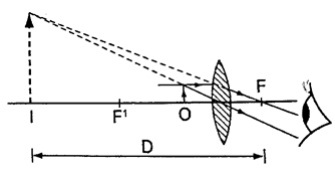Magnification in a simple microscope
Magnification produced depends on the focal length of the lens. Lens of short focal give greater magnification than those of long focal length. The angle ϐ subtended by the image at the eye is much greater than α which is the angle that the object would subtend at the eye when viewed without the lens. The ratio of the ϐ toα is known as angular magnification or magnifying power of an instrument. The angular magnification is equal to linear magnification.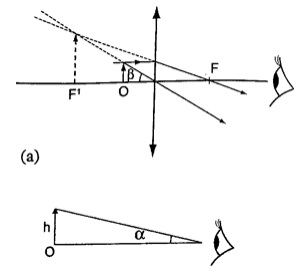Uses of a simple microscope
1. To study the features of small animals in biology
2. To look closely at small print on a map
3. To observe crystals in physics and chemistry
4. For forensic investigation by the police

#### 2. Compound microscope

It consists of two lenses with one nearer the object called the objective lens and the other nearer the eye called the eyepiece lens.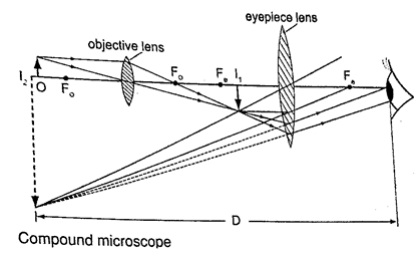Uses of compound microscope
1. Used to observe Brownian motion in science
2. To study micro-organisms and cells in biology
3. Analyze laboratory tests in hospital.

#### 4. The astronomical telescope

It is used to view distant stars. It consists of two lenses;
objective and eye-piece lenses. The objective lens has a large focal length while the eyepiece lens has a much shorter focal length.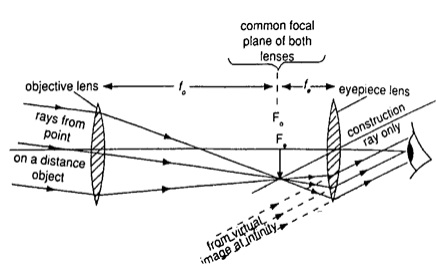#### 5. The camera

consists of a converging lens system, clicking button, shutter, diaphragm and a mounting base for the film all enclosed in a light proof box. The distance is adjusted to obtain a clear focus. The diaphragm has a hole called the aperture with an adjusting control knob to control the amount of light entering the camera. The shutter opens to allow light and close at a given time interval.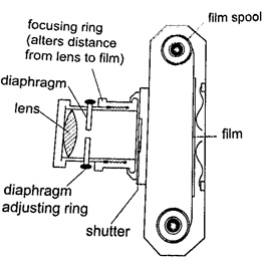Uses of a camera
1. The sine camera is used to make motion pictures
2. High speed cameras are used to record movement of particles
3. Close circuit television cameras (CCTV) are used to protect high security installations like banks, supermarkets etc.
4. Digital cameras are used to capture data that can be fed to computers.

#### 6. Human eye

It consists of a transparent cornea, aqueous humour and a crystal-like lens which form a converging lens system. The ciliary muscles contract or relax to change the curvature of the lens. Though the image formed at the retina is inverted the brain ‘sees’ the image as upright. For distant objects ciliary muscles relax while near objects it contracts to control the focal length and this is known as accommodation. When at 25 cmaway an object appears clearest and this is known as least distance of vision or near point.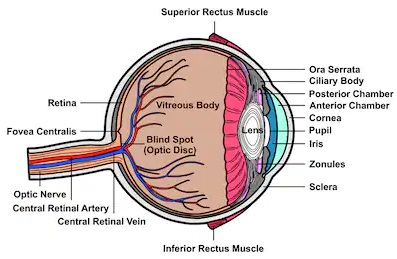### Common Eye Defects

#### 1. Short sightedness or hypermetropia

the eyeball is too large for the ‘relaxed focal length’ of the eye. The defect is corrected by placing a concave lensin front of the eye.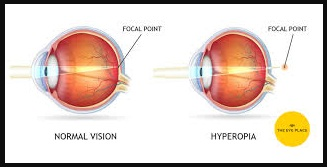#### 2. Long sightedness or myopia

images are formed beyond the retina. The defect is corrected by placing a converging lensin front of the eye.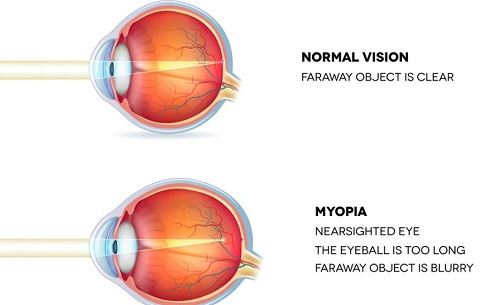#### 3. Presbyopia

this is the inability of the eye to accommodate and this occurs as the eye ages due to the weakening of the ciliary muscles. It can be corrected by the use a pair of spectacles.

#### 4. Astigmatism

this is a defect where the eye has two different focal lengths as a result of the cornea not being spherical. Corrected by the use of cylindrical lens.

#### 5. Colour blindness

caused by deficiency of colour detecting cells in the retina.

### Power of lens

The power of a simple lens is given by the formula: Power = 1 / f. The unit for power of a lens is diopter (D).

#### Example

Find the power of a concave lens of a focal length 25 cm.
Solution
Power = 1 /f = 1 / -0.25 = -4 D